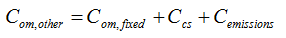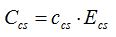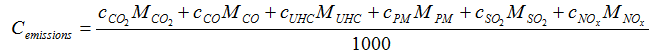# HOMER Pro 3.10

 Navigation: Glossary Other Operation and Maintenance CostType: Output Variable Units: \$/yr Symbol: Com,other

The other O&M cost is the sum of the system fixed O&M cost, the penalty for capacity shortage, and the penalties for emissions of pollutants.

HOMER uses the following equation to calculate the other O&M cost:where: Com,fixed Ccs = the penalty for capacity shortage [\$/yr] Cemissions = the penalty for emissions [\$/yr]

HOMER uses the following equation to calculate the penalty for capacity shortage:where: ccs = capacity shortage penalty [\$/kWh] Ecs = total capacity shortage [kWh/yr]

HOMER uses the following equation to calculate the penalty for emissions:where: cCO2 = penalty for emissions of CO2 [\$/t] cCO = penalty for emissions of CO [\$/t] cUHC = penalty for emissions of unburned hydrocarbons (UHC) [\$/t] cPM = penalty for emissions of particulate matter (PM) [\$/t] cSO2 = penalty for emissions of SO2 [\$/t] cNOx = penalty for emissions of NOx [\$/t] MCO2 = annual emissions of CO2 [kg/yr] MCO = annual emissions of CO [kg/yr] MUHC = annual emissions of unburned hydrocarbons (UHC) [kg/yr] MPM = annual emissions of particulate matter (PM) [kg/yr] MSO2 = annual emissions of SO2 [kg/yr] MNOx = annual emissions of NOx [kg/yr]

You can specify the penalties for each pollutant on the Emissions page. For information on how HOMER calculates the emissions of each pollutant, see the How HOMER calculates emissions section of the help.